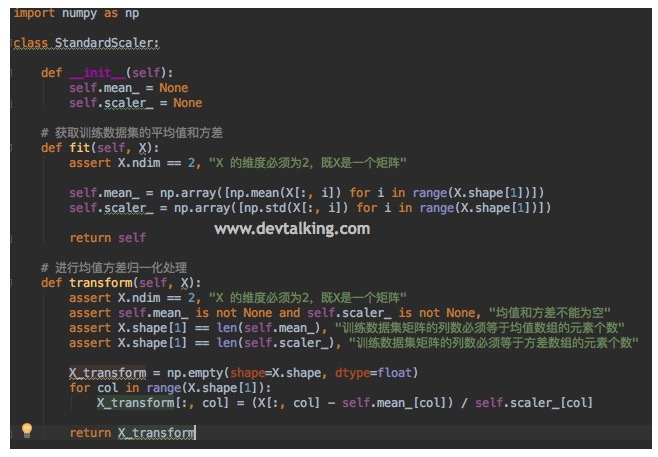## 封装kNN算法

PyCharm的配置这里我就不再累赘，如图所示，我们创建了一个Python文件kNN.py，然后定义了kNNClassify方法，该方法有4个参数，分别是kNN算法的k值，训练样本特征数据集XTrain，训练样本类别数据集yTrain，预测特征数据集x。该方法中的实现和在Jupyter Notebook中实现的一模一样，只不过加了三个断言，让方法的健壮性更好一点。我们给出N维欧拉定理

$$\sqrt {\sum_{i=1}^n(x_i^{(a)}-x_i^{(b)})^2}$$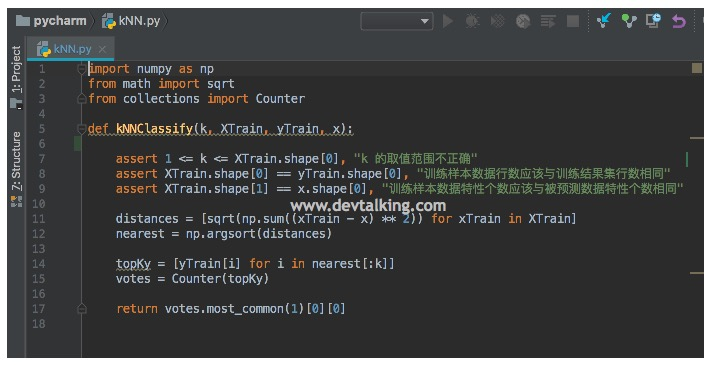## 机器学习流程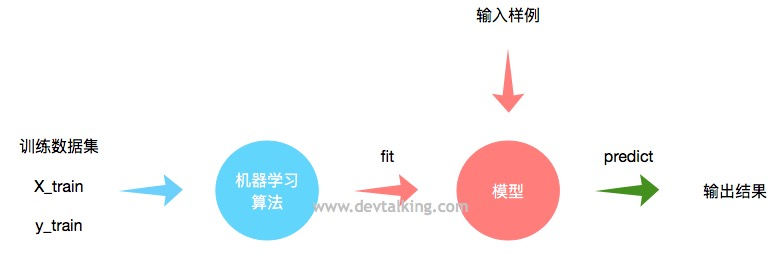## 重新封装kNN算法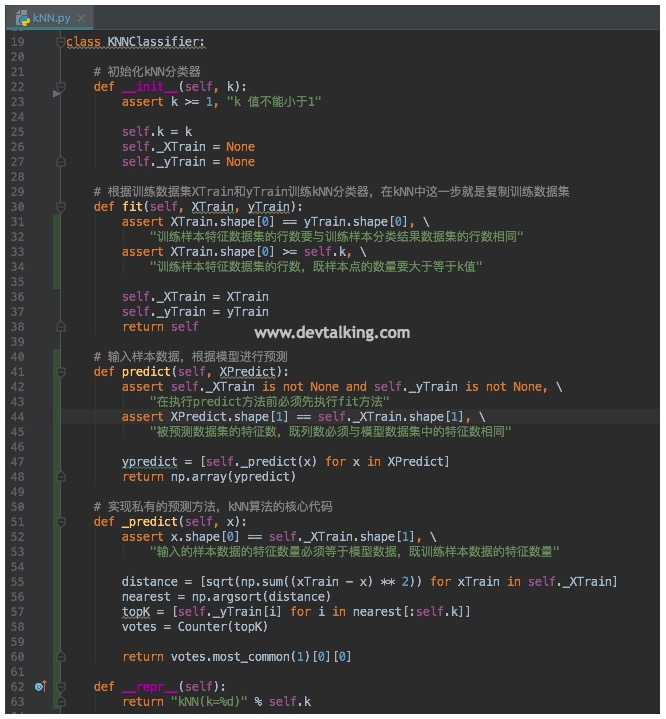## 判断机器学习算法的性能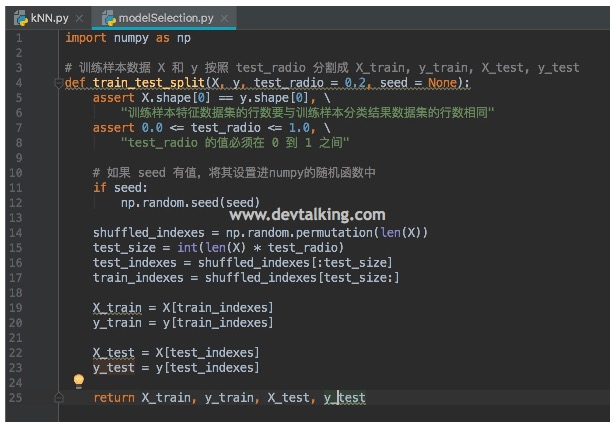• 首先train_test_split函数有四个参数，两个必填参数，两个非必填有默认值的参数。X是训练样本特征数据集，y是训练样本分类结果数据集，test_radio是设置训练数据和测试数据的比例，seed就很好理解了，就是NumPy的随机函数提供的随机种子机制。
• 上面代码中有一个方法大家之前应该没见过，那就是permutation(x)，该方法表示返回一个乱序的一维向量，元素从0到x，所以shuffled_indexes是一个乱序的一维向量数组，它的元素总数为训练样本数据的总数，既训练样本数据矩阵的行数，元素的范围从0到训练样本数据的总数。
• 根据test_radio计算出需要分割出的测试数据数量test_size
• 根据test_sizeshuffled_indexes中取出test_indexestrain_indexes，这两个数组中存的元素就是作为索引来用的。
• 根据test_indexestrain_indexesXy中得到X_trainy_trainX_testy_test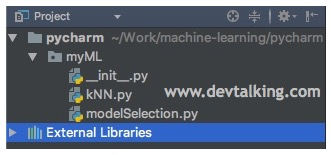## 超参数

### 寻找最好的k值

Scikit Learn中kNN算法的k值默认是5，有时候这个值并不是最优的值，那么我们可以通过一个简单的方式来寻找到最优的k值，那就是给定一个k值的范围，然后循环传入算法求训练分数最好的那个k值：

### 距离的权重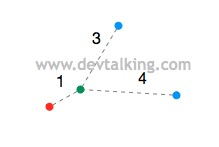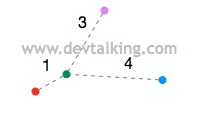### 距离的类型

$$\sum_{i=1}^n |X_i^{(a)}-X_i^{(b)}|$$

$$\sqrt {\sum_{i=1}^n(x_i^{(a)}-x_i^{(b)})^2} = \sqrt {\sum_{i=1}^n|x_i^{(a)}-x_i^{(b)}|^2} = (\sum_{i=1}^n|x_i^{(a)}-x_i^{(b)}|^2)^\frac 1 2$$

$$\sum_{i=1}^n |X_i^{(a)}-X_i^{(b)}| = （\sum_{i=1}^n |X_i^{(a)}-X_i^{(b)}|^1）^\frac 1 1$$

$$（\sum_{i=1}^n |X_i^{(a)}-X_i^{(b)}|^p）^\frac 1 p$$

## 网格搜索超参数

GridSearchCV也提供了几个属性，可以让我们方便的查看超参数和模型评分：

### GridSearchCV的其他参数

• n_jobs：该参数决定了在进行网格搜索时使用当前计算机的CPU核数，1就是使用1个核，2就是使用2个核，如果设置为-1，那么代表使用所有的核进行搜索。
• verbose：该参数决定了在网格搜索时的日志输出级别。

## 数据归一化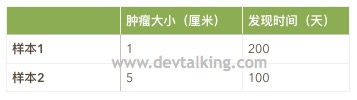$$x_{scale} = \frac {x - x_{min}} {x_{max} - x_{min}}$$

$$x_{scale} = \frac {x - x_{mean}} S$$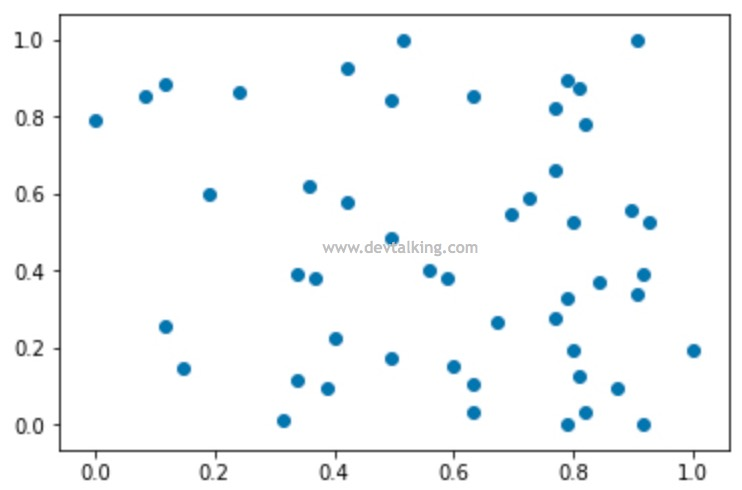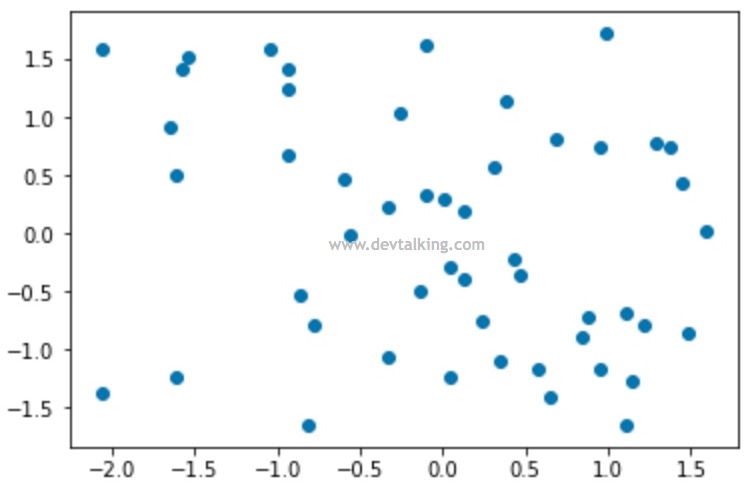## 如何对测试数据集进行归一化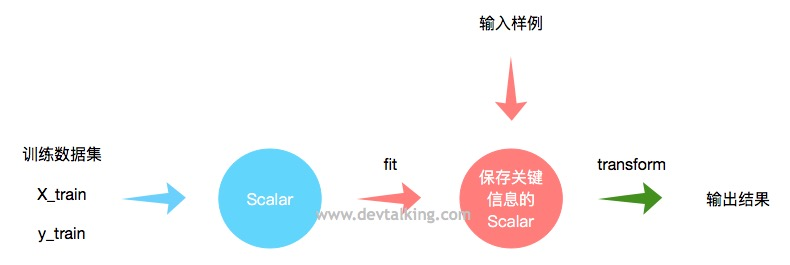Scalar的使用流程和机器学习算法的使用流程很像，输入训练数据集，进行fit操作，这里的fit操作就不是训练模型了，而是进行数据归一化处理，然后是transform，既对需要预测的数据进行归一化。我们来看看如何使用：

## 封装自己的数据归一化方法